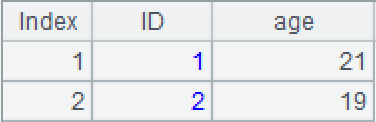icursor()

T.icursor( C,…;w,I )

T.icursor(C,…;w,I)

T为复组表时每个分表分别使用索引，不一定每个分表都有索引，没有则遍历。

 T 实表/复组表 C 实表中的列名，缺省时读取全部列 w 过滤条件，语法支持>、>=、<、<=、==、contain，过滤条件中用到的T表字段必须跟索引中的索引字段一致 I 索引名称，可省略

 @s 确保返回结果集对I有序且可支持大结果集 @u 从左到右处理多&&条件，缺省会根据条件优化过滤次序

 A 1 =file("D:\\emp1.ctx") 2 =A1.create(#EID,NAME,DEPT,GENER) 创建组表的基表 3 =demo.cursor("select EID,NAME,DEPT,GENDER from employee where EID< 100") 4 =A2.append(A3) 5 =A4.index(test_index,GENDER=="F";DEPT;EID) 创建索引test_index 6 =A4.icursor(;DEPT=="HR",test_index) 使用索引test_index查询出DEPT为HR的所有列的数据，结果返回游标 7 =A5.fetch() 获取游标中的数据8 =A4.icursor@u(;like(DEPT,"*ale*")&&EID>=50,test_index).fetch() 从左到右处理多&&条件，使用索引test_index查询含有“ale”的DEPT，并且EID大于等于50的数据9 =file("emp.ctx":[2,3]) 返回文件组 10 =A9.open() 打开复组表 11 =A10.index(index_cp,SALARY>10000;GENDER;) 为复组表创建索引index_cp 12 =A10.icursor(;GENDER=="F",index_cp).fetch() 使用索引index_cp查询SALARY为F的数据T.icursor()

T.icursor(C,…;w,I)

 T 集群表 C 集群表中的列名，缺省时读取全部列 w 过滤条件，语法支持>、>=、<、<=、==、contain，过滤条件中用到的T表字段必须跟索引中的索引字段一致 I 索引名称，可省略

 A 1 =file("emp1.ctx","192.168.0.101:8281") 打开集群文件 2 =A1.open() 打开集群表 3 =A2.attach(table1) 取出集群表table1 4 =A3.index(test_index2,GENDER=="F";DEPT;) 创建索引文件test_index2 5 =A3.icursor(;DEPT=="HR",test_index2) 使用索引test_index2查询出集群表A3中DEPT为HR所有列的数据，结果返回集群游标 6 =A5.fetch()7 =A2.index(test_index1,EID<16;NAME;) 创建索引文件test_index1 8 =A2.icursor(EID,SURNAME;NAME=="Smith",test_index1) 使用索引test_index1查询出集群表A2中NAME为Smith的EID、SURNAME列的数据，结果返回集群游标 9 =A8.fetch()